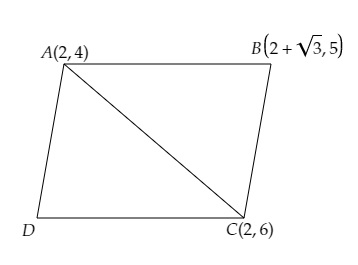# Find the area of a parallelogram $ABCD$ if three of its vertices are $A (2, 4), B (2 + \sqrt3, 5)$ and $C (2, 6)$.

Given:

Three vertices of a parallelogram are $A (2, 4), B (2 + \sqrt3, 5)$ and $C (2, 6)$.

To do:

We have to find the area of the parallelogram.

Solution:

Join $A$ and $C$ to get two triangles $ABC$ and $ADC$.We know that,

A diagonal bisects a triangle into two triangles of equal area.

This implies,

Area of parallelogram $ABCD=$ Area of triangle $ABC+$ Area of triangle $ADC$.

$=2\times$ Area of triangle $ABC$

We know that,

Area of a triangle with vertices $(x_1,y_1), (x_2,y_2), (x_3,y_3)$ is given by,

Area of $\Delta=\frac{1}{2}[x_{1}(y_{2}-y_{3})+x_{2}(y_{3}-y_{1})+x_{3}(y_{1}-y_{2})]$

Therefore,

Area of triangle $ABC=\frac{1}{2}[2(5-6)+(2+\sqrt3)(6-4)+2(4-5)]$

$=\frac{1}{2}[2(-1)+(2+\sqrt3)(2)+2(-1)]$

$=\frac{1}{2}[-2+4+2\sqrt3-2]$

$=\frac{1}{2} \times (2\sqrt3)$

$=\sqrt3$ sq. units.

Therefore,

The area of the parallelogram $ABCD=2\times \sqrt3=2\sqrt3$ sq. units.

The area of the parallelogram $ABCD$ is $2\sqrt3$ sq. units.

Updated on: 10-Oct-2022

48 Views